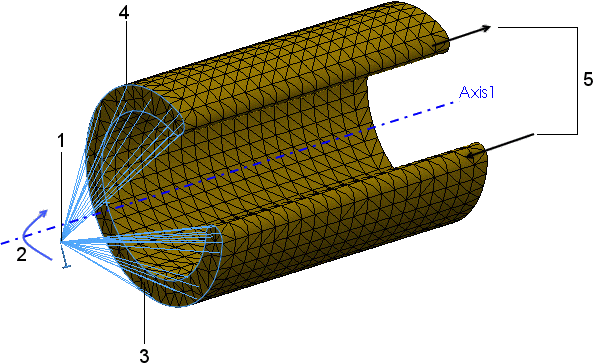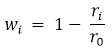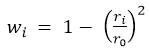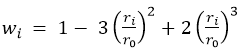Distributed Coupling for Remote Load and Mass

Distributed coupling constrains the motion of the coupling nodes to the translation and rotation of the reference node.

The distributed coupling constraint is enforced in an average sense in a way that enables control of the transmission of loads and displacements through weight factors at the coupling nodes. Distributed coupling allows the coupling nodes of the selected geometry (face or edge) to move relative to each other.Example of a Distributed Coupling Constraint
 1 Reference node 2 Prescribed rotation 3 Face that defines the coupling nodes 4 Coupling nodes 5 Warping (out of plane deformation) permitted by the distributed coupling constraint

Forces and moments at the reference node are distributed either as a coupling node-force distribution only (default) or as a coupling node-force and moment distribution. This constraint distributes loads such that the resultants of the forces (and moments) at the coupling nodes are equivalent to the forces and moments at the reference node.

For cases of more than a few coupling nodes, the distribution of remote forces/moments and mass is not determined by equilibrium alone, and distributing weight factors scale the distribution. These weighting factors are available when Connection Type is Distributed:
 Default (constant) Uniform weight distribution. All weight factors wi are equal to 1. Linear Weight factors decrease linearly with distance from the reference node.wi is the weight factor at coupling node i, ri is the coupling node radial distance from the reference node, and r0 is the distance to the furthest coupling node. Quadratic Weight factors decrease with distance from the reference node following a quadratic polynomial formulation.Cubic Weight factors decrease with distance from the reference node following a cubic polynomial formulation.When Connection Type is Rigid, the coupling nodes do not move relative to each other. Rigid bars connect the attachment point to the coupling nodes, and high stresses can develop as a result. The faces where remote loads or displacements are applied behave like rigid bodies.
The remote load and mass combinations supported for the Distributed and Rigid connections are listed below (Linear static and Nonlinear static studies).
Remote Load Combinations Distributed Connection Rigid Connection
Force and Moment Supported Supported
Translation and Rotation Supported Supported
Force, Moment, Translation, and Rotation Supported Not supported
Force, Moment, and Mass Supported Supported
Translation, Rotation, and Mass Supported Not supported
Force, Moment, Translation, Rotation, and Mass Supported Not supported
For Mass, you need to define Gravity (and perhaps Centrifugal load).